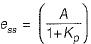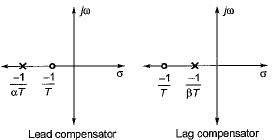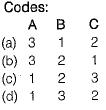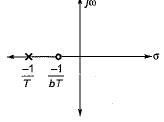Courses

# Industrial Controllers & Compensators

## 10 Questions MCQ Test Topicwise Question Bank for GATE Electrical Engineering | Industrial Controllers & Compensators

Description
This mock test of Industrial Controllers & Compensators for GATE helps you for every GATE entrance exam. This contains 10 Multiple Choice Questions for GATE Industrial Controllers & Compensators (mcq) to study with solutions a complete question bank. The solved questions answers in this Industrial Controllers & Compensators quiz give you a good mix of easy questions and tough questions. GATE students definitely take this Industrial Controllers & Compensators exercise for a better result in the exam. You can find other Industrial Controllers & Compensators extra questions, long questions & short questions for GATE on EduRev as well by searching above.
QUESTION: 1

### A first order dynamic linear system with a proportional controller exhibits an offset to a unit step input. The offset can be reduced by

Solution:

One of the disadvantage of a proportional controller is that it exhibits a permanent residual error at its operating point known as offset and
given byThe offset can be

QUESTION: 2

### Addition of zeros in a transfer function causes

Solution:

Pole-zero plot for lead and lag compensators are shown below.Since zero is close to origin in a lead compensator, therefore addition of zero in a transfer function will cause lead compensator.

QUESTION: 3

### A phase lead compensation network

Solution:
QUESTION: 4

Match List-I (Type of controllers) with List-II (Operation) and select the correct answer using the codes given below the lists:
List-I
A. Hydraulic controller
B. Pneumatic controller
C. Electronic controller
List-II
1. Fire and explosion of proof operation
2. Flexible operation
3. High torque, high speed operationSolution:
QUESTION: 5

Consider the following statements related to compensators used in control system applications:
1. When transient response is satisfactory and steady state characteristics are not satisfactory, normally lag compensation is employed.
2. Phase lag network is used to increase system stability and maintain velocity gain constant.
3. The capacitance is not used to fabricate a lag network.

Which of these statements is/are correct?

Solution:

Lag compensator is used to reduce steady-state error, therefore statement-1 is true.
As lag compensator reduces steady state error therefore, statement-2 is false.
Statement-3 is also false because capacitance is used to fabricate a lag network.

QUESTION: 6

The transfer function of a phase-lead controller is given by

Solution:

For lead compensator, zero is near to origin and pole is near to infinity as shown below. So, constant b will be more than unity and time constant T will be greater than zero.QUESTION: 7

The input to a controller is

Solution:

For any type of controller, the input is always an error signal.

QUESTION: 8

Addition of a pole to the closed-loop transfer function results in

Solution:
QUESTION: 9

Which of the following properties is/are not exhibited by a phase lead network?
1. The velocity constant is usually increased.
2. The slope of the magnitude curve is reduced at the gain cross over frequency.
3. The bandwidth is reduced.
4. The response becomes faster.

Solution:

Due to a phase lead network, bandwidth is increased. Hence, option (d) is correct.

QUESTION: 10

Assertion (A): If the phase shift decreases rapidly near the gain cross over frequency, phase lead compensation becomes ineffective. Reason
(R): The additional phase lead at gain crossover frequency is added to a much smaller phase angle than that at the old gain crossover frequency.

Solution: